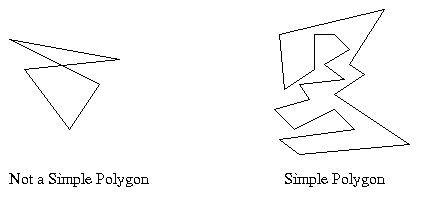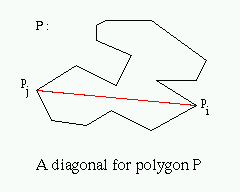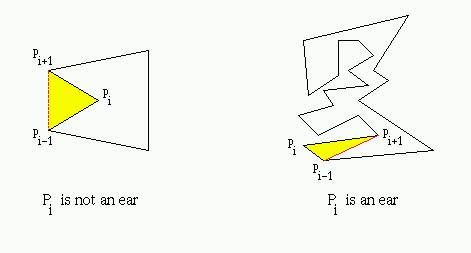Introduction to Ear Cutting for Simple Polygons

First of all, some very important definitions are introduced.

# Definition #1: Polygon

A polygon is a closed chain of n line segments (pi, pi+1) for 0 <= i <= n-1 and (pn-1, p0) , where n >= 3. The polygon P is represented by its vertices P = (p0, p1,..., pn-1).2 Polygons

# Definition #2: Simple Polygon

A simple polygon is a polygon P with no two non-consecutive edges intersecting. There is a well-defined bounded interior and an unbounded exterior for a simple polygon, where the interior is surrounded by edges. When referring to P, the convention is to include the interior of P.# Definition #3: Diagonal

A diagonal is a line segment lying entirely inside polygon P and joining two non-consecutive vertices pi and pj# Definition #4: Principal Vertex

A vertex pi is called a principal vertex if the diagonal (pi-1, pi+1) intersects the boundary of simple polygon P only at pi-1 and pi+1 .# Definition #5: Ear

A principal vertex pi of a simple polygon P is called an ear if the diagonal (pi-1, pi+1) that bridges pi lies entirely in P. We say that two ears pi and pj are non-overlapping if the interior of triangle (pi-1, pi, pi+1) does not intersect the interior of triangle (pj-1, pj, pj+1) .